ISRO-2018

 Question 1
The difference between a named pipe and a regular file in Unix is that
 A Unlike a regular file, named pipe is a special file B The data in a pipe is transient, unlike the content of a regular file C Pipes forbid random accessing, while regular files do allow this. D All of the above
Operating-Systems       UNIX-Operating-System
Question 1 Explanation:
→ Named pipe is a special instance of a file that has no contents on the filesystem.
→ A FIFO special file (a named pipe) is similar to a pipe, except that it is accessed as part of the filesystem. It can be opened by multiple processes for reading or writing. When processes are exchanging data via the FIFO, the kernel passes all data internally without writing it to the filesystem. Thus, the FIFO special file has no contents on the filesystem; the filesystem entry merely serves as a reference point so that processes can access the pipe using a name in the filesystem.
→ The kernel maintains exactly one pipe object for each FIFO special file that is opened by at least one process. The FIFO must be opened on both ends (reading and writing) before data can be passed. Normally, opening the FIFO blocks until the other end is opened also.
 Question 2
A class of 30 students occupy a classroom containing 5 rows of seats, with 8 seats in each row. If the students seat themselves at random, the probability that the sixth seat in the fifth row will be empty is
 A 1/5 B 1/3 C 1/4 D 2/5
Engineering-Mathematics       Probability
Question 2 Explanation:
Step-1: Given, 5 rows with 8 seats in each row.
Total number of seats = 5*40
= 40 seats
Total number of students= 30
Step-2: Given constraint that, 6th seat in the fifth row is empty
When we are deleting 6th seat, 30 students have 39 choices of seats
Step-3: Total number of choices = 39C30
Total ways to choose = 40C30
Step-4: Final probability = 39C30 / 40C30
= 1/4
 Question 3
The domain of the function log( log sin(x) ) is
 A 0 < x < π B 2nπ < x < (2n+1)π , for n in N C Empty set D None of the above
Engineering-Mathematics       Calculus
Question 3 Explanation:
→ The range of sinx value lies between -1 and 1 and whereas log(sinx) value will be the positive value.
→ So the domain of log(log sin(x)) is undefined which is empty Set.
 Question 4
The following paradigm can be used to find the solution of the problem in minimum time: Given a set of non-negative integer, and a value K, determine if there is a subset of the given set with the sum equal to K:
 A Divide and Conquer B Dynamic Programming C Greedy Algorithm D Branch and Bound
Algorithms       Dynamic-Programming
Question 4 Explanation:
The above problem clearly specifies that sum of subset problem. The sum of the subset problem using recursion will give exponential time. Using dynamic programming we can get pseudo-polynomial time.
Sum of subset problem:
Given a set of non-negative integers, and a value sum, determine if there is a subset of the given set with the sum equal to the given sum.

Example:
Array set[ ]= {1,2,3,4,5} and sum=6
Here there are possible subsets (2,4),(1,2,3) and (5,1).
 Question 5
( G, *) is an abelian group. Then
 A x = x-1, for any x belonging to G B x = x2, for any x belonging to G C (x * y )2 = x2 * y2, for any x, y belonging to G D G is of finite order
Engineering-Mathematics       Set-Theory
Question 5 Explanation:
An abelian group is a commutative group. As per the above options, option C is the correct answer because it follows commutative property.
(x * y )2 = x2 * y2, for any x, y belonging to G
 Question 6
Consider the following C code segment:For the program fragment above, which of the following statements about the variables i and j must be true after the execution of this program? [!(exclamation) sign denotes factorial in the Solution]
 A ( j=(x-1)!) ∧ (i>=x) B ( j = 9!) ∧ (i =10) C (( j = 10!) ∧ (i = 10 )) V (( j = (x - 1)!) ∧ (i = x )) D (( j = 9!) ∧ (i = 10)) V (( j = (x - 1)!) ∧ (i = x ))
Programming-for-Output-Problems       Control Flow
Question 6 Explanation:
As per code segment,
Step-1: The ith loop will compute from value 1 to 9. If the condition is true, then it computes j=j*i statement.
Step-2: The statement j=j*i is nothing but calculating 9!. If the condition fails automatically while loop will be terminated. It means maximum we can execute 9 values.
Step-3: when x becomes 10 then maximum I value will be 9. So, we can calculate up to 9! And I become 10 then it terminates the condition.
 Question 7
A computer uses ternary system instead of the traditional binary system. An n bit string in the binary system will occupy
 A 3+n ternary digits B 2n/3 ternary digits C n(log23) ternary digits D n(log32 ) ternary digits
Digital-Logic-Design       Number-Systems
Question 7 Explanation:
→ Binary numbers are maximum 2n-1.
→ But in question they are given ternary numbers, it means 3x-1.
→ Both will take different no. of bits to represent the same number.
3x -1 = 2n -1
3x = 2n
Apply log on both side
x= log3( 2n)
x=n*log32 .
 Question 8
Which of the following is application of Breadth First Search on the graph?
 A Finding the diameter of the graph B Finding the bipartite graph C Both (a) and (b) D None of the above
Data-Structures       Graphs
Question 8 Explanation:
Breadth-first search can be used to solve many problems in graph theory

Examples
1. Copying garbage collection, Cheney's algorithm
2. Find the diameter of the graph
3. Testing bipartiteness of a graph.
4. Finding the shortest path between two nodes u and v, with path length measured by the number of edges (an advantage over depth-first search)
5. (Reverse) Cuthill–McKee mesh numbering
6. Ford–Fulkerson method for computing the maximum flow in a flow network
7. Serialization/Deserialization of a binary tree vs serialization in sorted order, allows the tree to be reconstructed in an efficient manner.
8. Construction of the failure function of the Aho-Corasick pattern matcher.
 Question 9
Micro program is
 A The name of a source program in microcomputers B Set of micro instructions that define the individual operations in response to a machine-language instruction C A primitive form of macros used in assembly language programming D A very small segment of machine code
Computer-Organization
Question 9 Explanation:
→ The Microprogram is computing a sequence of microinstructions that controls the operation of an arithmetic and logic unit so that machine code instructions are executed.
→ The Microprogram is set of microinstructions that define the individual operations in response to a machine-language instruction
 Question 10
Given two sorted list of size m and n respectively. The number of comparisons needed the worst case by the merge sort algorithm will be
 A m*n B maximum of m and n C minimum of m and n D m+n–1
Algorithms       Sorting
Question 10 Explanation:
Here the maximum number of comparisons is m+n-1.
For example: Take 2 sub-arrays of size 3 such as 1,3,5 and another subarray 2,4,6. Then in this case number of comparisons is 5 which is m+n-1 can be taken as O(m+n).
 Question 11
In unit testing of a module, it is found that for a set of test data, at the maximum 90% of the code alone were tested with the probability of success 0.9. The reliability of the module is
 A Greater than 0.9 B Equal to 0.9 C At most 0.81 D At least 0.81
Software-Engineering       Software-testing
Question 11 Explanation:
Given data
Step-1: 90% of code were tested,
Testing with the probability=0.9
Step-2: We can write tested data into 0.9 because it is given in percentages.
Step-3: Reliability of the module = Tested data * probability
= 0.9 * 0.9
= 0.81(at most)
 Question 12
In a file which contains 1 million records and the order of the tree is 100, then what is the maximum number of nodes to be accessed if B+ tree index is used?
 A 5 B 4 C 3 D 10
Database-Management-System       B-and-B+-Trees
Question 12 Explanation:
Explanation:
→ If there are K search-key values in the file, the path is no longer than ⌈log⌈n/2⌉(K)⌉.
→ A node is generally the same size as a disk block, typically 4 kilobytes, and n is typically around 100 (40 bytes per index entry).
→ With 1 million search key values and n = 100, at most log50(1000000)=4 nodes are accessed in a lookup.
Note: Contrast this with a balanced binary free with 1 million search key values around 20 nodes are accessed in a lookup. Above difference is significant since every node access may need a disk I/O, costing around 20 milliseconds!
 Question 13
A particular disk unit uses a bit string to record the occupancy or vacancy of its tracks, with 0 denoting vacant and 1 for occupied. A 32-bit segment of this string has hexadecimal value D4FE2003. The percentage of occupied tracks for the corresponding part of the disk, to the nearest percentage is
 A 12 B 25 C 38 D 44
Computer-Organization       Secondary-Memory
 Question 14
Which of the following is a dense index?
 A Primary index B Clusters index C Secondary index D secondary non-key index
Database-Management-System       B-and-B+-Trees
Question 14 Explanation:
→ The primary index is created for the primary key of a table. So, records are usually clustered according to the primary key. It can be sparse.
→ In the sparse index, index records are not created for every search key. An index record here contains a search key and an actual pointer to the data on the disk. To search a record, we first proceed by index record and reach the actual location of the data. If the data we are looking for is not where we directly reach by following the index, then the system starts a sequential search until the desired data is found.
→ The secondary index usually dense.
→ In the dense index, there is an index record for every search key value in the database. This makes searching faster but requires more space to store index records itself. Index records contain search key value and a pointer to the actual record on the disk.
 Question 15
In E-R model, Y is the dominant entity and X is a subordinate entity
 A If X is deleted, then Y is also deleted B If Y is deleted, then X is also deleted C If Y is deleted, then X is not deleted D None of the above
Database-Management-System       ER-Model
Question 15 Explanation:
→ It is the best example of referential integrity. In referential integrity, we are using a foreign key.
→ If we want to delete any entity in the dominant entity(Like primary key of a table) then subordinate entity(derived attribute) also deleted.
Note: If we want to delete any subordinate entity in entity then the dominant entity is not going to delete.
 Question 16
Of the following, which best characterizes computers that use memory-mapped I/O?
 A The computer provides special instructions for manipulating I/O ports B I/O ports are placed at addresses on the bus and are accessed just like other memory locations C To perform I/O operations, it is sufficient to place the data in an address register and call channel to perform the operation D I/O can be performed only when memory management hardware is turned on
Computer-Organization       Hardware Devices
Question 16 Explanation:
→ Memory-mapped I/O uses the same address space to address both memory and I/O devices.
→ The memory and registers of the I/O devices are mapped to (associated with) address values.
→ So when an address is accessed by the CPU, it may refer to a portion of physical RAM, or it can instead refer to the memory of the I/O device. Thus, the CPU instructions used to access the memory can also be used for accessing devices.
→ Each I/O device monitors the CPU's address bus and responds to any CPU access of an address assigned to that device, connecting the data bus to the desired device's hardware register.
→ To accommodate the I/O devices, areas of the addresses used by the CPU must be reserved for I/O and must not be available for normal physical memory.
→ The reservation may be permanent, or temporary (as achieved via bank switching).
 Question 17
Of the following sorting algorithms, which has a running time that is least dependent on the initial ordering of the input?
 A Merge Sort B Insertion Sort C Selection Sort D Quick Sort
Algorithms       Sorting
Question 17 Explanation:Question 18
Processes P1 and P2 have a producer-consumer relationship, communicating by the use of a set of shared buffers.Increasing the number of buffers is likely to do which of the following? I. Increase the rate at which requests are satisfied (throughput) II. Decrease the likelihood of deadlock III. Increase the ease of achieving a correct implementation
 A III Only B II Only C I Only D II and III Only
Question 18 Explanation:
It only satisfied statement I. because increasing the memory size increases the rate at which requests are satisfied but can not alter the possibility of deadlock and neither does it play any role in implementation.
 Question 19
A doubly linked list is declared asWhere Fwd and Bwd represent forward and a backward link to the adjacent elements of the list. Which of the following segments of code deletes the node pointed to by X from the doubly linked list, if it is assumed that X points to neither the first nor the last node of the list?
 A X→ Bwd→ Fwd = X→ Fwd; X→ Fwd → Bwd = X→ Bwd ; B X→ Bwd.Fwd = X→ Fwd ; X.Fwd→ Bwd = X→ Bwd ; C X.Bwd→ Fwd = X.Bwd ; X→ Fwd.Bwd = X.Bwd ; D X→ Bwd→ Fwd = X→ Bwd ; X→ Fwd→ Bwd = X→ Fwd;
Question 19 Explanation:
Let the element just before x be y and that just after be z. In order to link y to z,
we need x→ Bwd→ Fwd = x.
Fwd and in order to link z to y, we need x→ Fwd→ Bwd=x→ Bwd.
 Question 20
If Tree-1 and Tree-2 are the trees indicated below :Which traversals of Tree-1 and Tree-2, respectively, will produce the same sequence?
 A Preorder, Postorder B Postorder, Inorder C Postorder, Preorder D Inorder, Preorder
Data-Structures       Binary-Trees
Question 20 Explanation:
→ Pre order traverses Root, Left, Right.
→ Post order traverses Left, Right, Root.
→ In-order traverses Left, Root, Right.
Tree-1: Post order: GJIHEFBDCA
Tree-2: In order: GJIHEFBDCA
 Question 21
In the diagram above, the inverter (NOT gate) and the AND-gates labelled 1 and 2 have delays of 9, 10 and 12 nanoseconds(ns), respectively. Wire delays are negligible. For certain values of a and c, together with the certain transition of b, a glitch (spurious output) is generated for a short time, after which the output assumes its correct value. The duration of the glitch isA 7ns B 9ns C 11ns D 13ns
Digital-Logic-Design       Logic-Gates
Question 21 Explanation:
Step-1: Inverter→ NOT gate(b’) will generate 9ns
Step-2: 1st AND gate(ab’) takes 19ns because combining NOT gate(9ns)+extra(10ns)
Step-3: Second AND gate(bc) takes only 12ns because we are not using inverter here.
Step-4: A glitch will take 1st AND gate(ab’) - Second AND gate(bc)
=19-12
=7ns
 Question 22
Which of the following comparisons between static and dynamic type checking is incorrect?
 A Dynamic type checking slows down the execution B Dynamic type checking offers more flexibility to the programmers C In contrast to Static type checking, dynamic type checking may cause failure in runtime due to type errors D Unlike static type checking, dynamic type checking is done during compilation
Compiler-Design       Compilers
Question 22 Explanation:
→ Type checking is the process of verifying and enforcing the constraints of types, and it can occur either at compile time (i.e. statically) or at runtime (i.e. dynamically).
→ Type checking is all about ensuring that the program is type-safe, meaning that the possibility of type errors is kept to a minimum.
→ A language is statically-typed if the type of a variable is known at compile time instead of at runtime. Common examples of statically-typed languages include Ada, C, C++, C#, JADE, Java, Fortran, Haskell, ML, Pascal, and Scala.
→ Dynamic type checking is the process of verifying the type safety of a program at runtime. Common dynamically-typed languages include Groovy, JavaScript, Lisp, Lua, Objective-C, PHP, Prolog, Python, Ruby, Smalltalk and Tcl.
 Question 23
_______ can detect burst error of length less than or equal to degree of the polynomial and detects burst errors that affect odd number of bits.
 A Hamming Code B CRC C VRC D None of the above
Computer-Networks       CRC
Question 23 Explanation:
→ A cyclic redundancy check (CRC) is an error-detecting code commonly used in digital networks and storage devices to detect accidental changes to raw data. CRCs can be used for error correction
→ The design of the CRC polynomial depends on the maximum total length of the block to be protected (data + CRC bits), the desired error protection features, and the type of resources for implementing the CRC, as well as the desired performance.
→ A common misconception is that the "best" CRC polynomials are derived from either irreducible polynomials or irreducible polynomials times the factor 1 + x, which adds to the code the ability to detect all errors affecting an odd number of bits.
→ If we use the generator polynomial g(x)=p(x)(1+x), where p(x) is a primitive polynomial of degree r−1, then the maximal total block length is 2r−1 −1 , and the code is able to detect single, double, triple and an odd number of errors.
 Question 24
Station A uses 32-byte packets to transmit messages to Station B using a sliding window protocol. The round trip delay between A and B is 80 ms and the bottleneck bandwidth on the path between A and B is 128 kbps. What is the optimal window size that A should use?
 A 20 B 40 C 160 D 320
Computer-Networks       Control-Flow-Methods
Question 24 Explanation:
Tt = L / B = 32 bytes/ 128 kbps = 32*8/128 ms = 2 ms
Round trip delay = 2 * Tp = 80 ms (given)
Optimal window size is = (Tt + 2*Tp) / Tt = 82 / 2 = 41
Option is not given, closest option is 40.
 Question 25
Assuming that for a given network layer implementation, connection establishment overhead is 100 bytes and disconnection overhead is 28 bytes. What would be the minimum size of the packet the transport layer needs to keep up if it wishes to implement a datagram service above the network needs to keep its overhead to a minimum of 12.5%. (Ignore transport layer overhead)
 A 512 bytes B 768 bytes C 1152 bytes D 1024 bytes
Computer-Networks       Switching
Question 25 Explanation:
→ Given a question is based on circuit-switching.
→ In circuit-switching, the total time required to send data = Setup connection time + Transfer time + Teardown the connection time
→ In datagram-service, the total time required to send data = N*Setup connection time + Transfer time
 Question 26
In cryptography, the following uses transposition cyphers and the keyword is LAYER. Encrypt the following message. (Spaces are omitted during encryption) WELCOME TO NETWORK SECURITY!
 A WMEKREETSILTWETCOOCYONRU! B EETSICOOCYWMEKRONRU!LTWET C LTWETONRU!WMEKRCOOCYEETSI D ONRU!COOCYLTWETEETSIWMEKR
Computer-Networks       Network-Security
Question 26 Explanation:Question 27
In a particular program, it is found that 1% of the code accounts for 50% of the execution time. To code a program in C++, it takes 100 man-days. Coding in assembly language is 10 times harder than coding in C++ but runs 5 times faster. Converting an existing C++ program into an assembly language program is 4 times faster. To completely write the program in C++ and rewrite the 1% code in assembly language, if a project team needs 13 days, the team consists
 A 13 programmers B 10 programmers C 8 programmers D 100/13 programmers
C++
Question 27 Explanation:Question 28
Incremental Compiler is a compiler
 A which is written in a language that is different from the source language B compiles the whole source code to generate object code afresh C compiles only those portion of source code that has been modified. D that runs on one machine but produces object code for another machine
Compiler-Design       Compilers
Question 28 Explanation:
Types of compilers

1. Incremental compiler: It rebuilds all program modules, incremental compiler re-compiles only those portions of a program that have been modified.
2. Cross-compiler: If the compiled program can run on a computer whose CPU or operating system is different from the one on which the compiler runs, the compiler is a cross-compiler.
3. A bootstrap compiler: is written in the language that it intends to compile. A program that translates from a low-level language to a higher level one is a decompiler.
4. Source-to-source compiler or transpiler: A program that translates between high-level languages is usually called a source-to-source compiler or transpiler.
 Question 29
DU-chains(Definition-Use) in compiler design
 A Consist of a definition of a variable and all its uses, reachable from that definition B Are created using a form of static code analysis C Are prerequisite for many compiler optimization including constant propagation and common sub-expression elimination D All of the above
Compiler-Design       Compilers
Question 29 Explanation:
→ A Use-Definition Chain (UD Chain) is a data structure that consists of a use, U, of a variable, and all the definitions, D, of that variable that can reach that use without any other intervening definitions. A definition can have many forms but is generally taken to mean the assignment of some value to a variable (which is different from the use of the term that refers to the language construct involving a data type and allocating storage).
→ A counterpart of a UD Chain is a Definition-Use Chain (DU Chain), which consists of a definition, D, of a variable and all the uses, U, reachable from that definition without any other intervening definitions.
→ Both UD and DU chains are created by using a form of static code analysis known as data flow analysis. Knowing the use-def and def-use chains for a program or subprogram is a prerequisite for many compiler optimizations, including constant propagation and common subexpression elimination.
 Question 30
Which of the following comment about peephole optimization is true?
 A It is applied to a small part of the code and applied repeatedly B It can be used to optimize intermediate code C It can be applied to a portion of the code that is not contiguous D It is applied in the symbol table to optimize the memory requirements.
Compiler-Design       Code-Optimization
Question 30 Explanation:
→ Peephole optimization is a kind of optimization performed over a very small set of instructions in a segment of generated code. The set is called a "peephole" or a "window". It works by recognizing sets of instructions that can be replaced by shorter or faster sets of instructions.

Replacement Rules:
1. Null sequences – Delete useless operations.
2. Combine operations – Replace several operations with one equivalent.
3. Algebraic laws – Use algebraic laws to simplify or reorder instructions.
4. Special case instructions – Use instructions designed for special operand cases.
5. Address mode operations – Use address modes to simplify code.
 Question 31
A byte addressable computer has a memory capacity of 2m KB( kbytes ) and can perform 2n operations. An instruction involving 3 operands and one operator needs maximum of
 A 3m bits B 3m + n bits C m + n bits D None of the above
Computer-Organization
Question 31 Explanation:
Step-1: Memory capacity is 2m KB = 230m Bytes
Step-2: Total number of operations 2n
Step-3: Every instruction involving 3 operands and 1 operator
Step-4: Number of bits needed by instruction is= 3*(m+10)+n
=3m+n+30 bits
 Question 32
Any set of Boolean operators that is sufficient to represent all Boolean expressions is said to be complete. Which of the following is not complete?
 A { AND, OR } B { AND, NOT } C { NOT, OR } D { NOR }
Digital-Logic-Design       Boolean-Algebra
Question 32 Explanation:
→ NOT, AND and OR gates are basic gates
→ NAND and NOR gates are universal gates.
→ With the help of universal gates, we can construct any boolean expressions. These gates are also called functionally complete.
→ AND+NOT=NAND→ Functionally Complete
→ OR+NOT=NOR→ Functionally Complete
→ NOR→ Functionally Complete
→ AND+OR→ Not functionally complete
 Question 33
Consider a singly linked list of the form where F is a pointer to the first element in the linked list and L is the pointer to the last element in the list.The time of which of the following operations depends on the length of the list?
 A Delete the last element of the list B Delete the first element of the list C Add an element after the last element of the list D Interchange the first two elements of the list
Question 33 Explanation:
F and L must point to the first and last elements respectively.
Option B: It needs only the operation F=F→ next
Option C: It needs only the operations L→ next=new node, L = new node
Option D: It needs only the operations T=F, F=F→ next, T→ next =F→ next, F→ next=T
→ All these operations do not depend on the length of the list.
→ Indeed in order to delete the last element from the list, we need to first locate the element before the last (which can not be accessed from L). Thus we must parse all the list from the first and till the element just before the last after which we can delete the last element and assign L to the one before.
 Question 34
A particular BNF definition for a “word” is given by the following rules. <word> ::= <letter> | <letter><pairlet> | <letter><pairdig> <pairlet> ::= <letter><letter> | <pairlet><letter><letter> <pairdig> ::= <digit><digit> | <pairdig><digit><digit> <letter> ::= a | b | c | ... | y | | z <digit> ::= 0 | 1 | 2 | ... | 9 Which of the following lexical entries can be derived from < word > ? I. pick II. picks III. c44
 A I, II and III B I and II only C l and III only D lI and III only
Database-Management-System
Question 34 Explanation:
Step-1: Both < letter > and < digit > produce only a single character
Step-2: Both < pairlet > and < pairdig > produce even number of characters
Step-3: < word > produces odd number of characters.
Step-4: As per the statements I, II and III. I statement having even number of characters
So, a statement I is wrong. Statement II and III are having odd number of characters.
 Question 35
Avalanche effect in cryptography
 A Is desirable property of the cryptographic algorithm B Is undesirable property of the cryptographic algorithm C Has no effect on the encryption algorithm D None of the above
Computer-Networks       Network-Security
Question 35 Explanation:
→ In cryptography, the avalanche effect is the desirable property of cryptographic algorithms, typically block cyphers and cryptographic hash functions, wherein if input is changed slightly (for example, flipping a single bit), the output changes significantly (e.g., half the output bits flip).
→ In the case of high-quality block cyphers, such a small change in either the key or the plaintext should cause a drastic change in the ciphertext.
 Question 36
In neural network, the network capacity is defined as
 A The traffic carry capacity of the network B The total number of nodes in the network C The number of patterns that can be stored and recalled in a network D None of the above
Question 36 Explanation:
In neural network, the network capacity is defined as the number of patterns that can be stored and recalled in a network
 Question 37
Cloaking is a search engine optimization (SEO) technique. During cloaking
 A Content presented to search engine spider is different from that presented to the user’s browser B Content present to search engine spider and browser is the same C Contents of the user’s requested website are changed D None of the above
Question 37 Explanation:
→ Cloaking takes a user to other sites than he or she expects by disguising those sites' true content.
→ During cloaking, the search engine spider and the browser are presented with different content for the same Web page.
→ HTTP header information or IP addresses assist in sending the wrong Web pages.
→ Searchers will then access websites that contain information they simply were not seeking, including pornographic sites.
→ Website directories also offer up their share of cloaking techniques.
 Question 38
What is one advantage of setting up a DMZ(Demilitarized Zone) with two firewalls?
 A You can control where traffic goes in the three networks B You can do stateful packet filtering C You can do load balancing D Improve network performance
Computer-Networks       Network-Security
Question 38 Explanation:
→ The most secure approach is to use two firewalls to create a DMZ.
1. The first firewall (also called the "front-end" or "perimeter" firewall) must be configured to allow traffic destined to the DMZ only.
2. The second firewall (also called "back-end" or "internal" firewall) only allows traffic from the DMZ to the internal network.

This setup is considered more secure since two devices would need to be compromised. There is even more protection if the two firewalls are provided by two different vendors because it makes it less likely that both devices suffer from the same security vulnerabilities.
 Question 39
Which one of the following algorithm is not used in asymmetric key cryptography?
 A RSA Algorithm B Diffie-Hellman Algorithm C Electronic Code Book Algorithm D None of the above
Computer-Networks       Network-Security
Question 39 Explanation: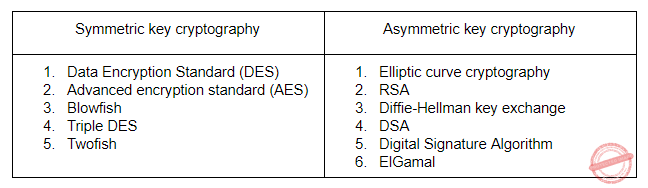Question 40
Consider the following table in a relational databaseA {Last Name} B {Room} C {Shift} D {Room, Shift}
Database-Management-System       Relational-databases
Question 40 Explanation:
→ Candidate means uniquely identified key.
→ In the above table, Room having duplicate values. So, we can’t say room is candidate key
→ The last name also having duplicates. So, we can’t say the last name is candidate key.
→ Shift also having duplicate keys, so we can’t say shift also a candidate key.
→ Combining Room and Shift we can say that candidate key.
 Question 41
A data driven machine is one that executes an instruction if the needed data is available. The physical ordering of the code listing does not dictate the course of execution. Consider the following pseudo-code: (A) Multiply E by 0.5 to get F (B) Add A and B to get E (C) Add B with 0.5 to get D (D) Add E and F to get G (E) Add A with 10.5 to get C Assume A, B, C are already assigned values and the desired output is G. Which of the following sequence of execution is valid?
 A B, C, D, A, E B C, B, E, A, D C A, B, C, D, E D E, D, C, B, A
Question 41 Explanation:
Given data,
A) F= E * 0.5
B) E=A+B
C) D=B+0.5
D) G=E+F
E) C=A+10.5
It is given A, B, C are already assigned values, so we can perform any of B or C or E first but not A. so option(c) is incorrect .
Option(a) is incorrect because we can't perform operation D before operation A.
Option(d) is incorrect because we can't perform operation D before operation A.
Hence option(b) is only correct.
 Question 42
Assume A and B are non zero positive integers. The following code segment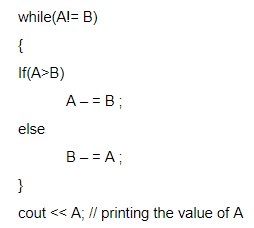A Computes the LCM of two numbers B Divides the larger number by the smaller number C Computes the GCD of two numbers D Finds the smaller of two numbers
Programming-for-Output-Problems       Control Flow
Question 42 Explanation:
→ The above iterative code is computes the GCD of two numbers using euclidean algorithm.
→ The procedure is to subtract smaller number from larger. So that we can reduce larger number then doesn’t change the value of GCD.
→ if we performing number of iterations based on condition the larger of two numbers will end up with GCD.
 Question 43
A language with string manipulation facilities uses the following operations.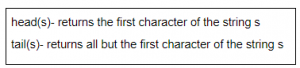concat(sl, s2)- concatenates string s1 with s2. The output of concat(head(s), head(tail(tail(s)))), where s is acbc is
 A ab B ba C ac D as
Theory-of-Computation       Languages-and-Grammars
Question 43 Explanation:Question 44
Choose the correct statement:
 A A = {an bn | n = 1, 2, 3, …. } is a regular language B The set B, consisting of all strings made up of only a’s and b’s having an equal number of a’s and b’s defines a regular language C L(A * B) ∩ B gives the set A D None of the above
Theory-of-Computation       Regular-Language
Question 44 Explanation:
Option A: False: A = {an bn | n=1,2,3,…. } is Deterministic Context Free Language(DCFL) but not regular.
Option B: False: Equal number of a's and equal number of b's is also DCFL but not regular
Option C: False: L(A*B) ∩ B gives the set B but not set A.
L(A*B)= {AB+ AAB + …+AAAAB+B}
L(A*B)∩B=B
 Question 45
CFG (Context Free Grammar) is not closed under
 A Union B Complementation C Kleene star D Product
Theory-of-Computation       Context-Free-Language
Question 45 Explanation:
CFG (Context Free Grammar) is not closed under complementation
 Question 46
The FSM (Finite State Machine) machine pictured in the figure below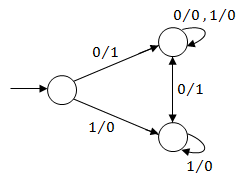A Complements a given bit pattern B Finds 2’s complement of a given bit pattern C Increments a given bit pattern by 1 D Changes the sign bit
Theory-of-Computation       Finite-Automata
Question 46 Explanation:
Let consider an example string:
Input = 1000
Output = 1111
The FSM, doesn't change until the first 1 come
I/p = 1000
1's complement = 0111
2's complement = 0111
---------------------------
1000 = I/p
----------------------------
It results 2's complement.
 Question 47
A CFG(Context Free Grammar) is said to be in Chomsky Normal Form (CNF), if all the productions are of the form A→ BC or A→ a. Let G be a CFG in CNF. To derive a string of terminals of length x, the number of products to be used is
 A 2x – 1 B 2x C 2x + 1 D 2x
Theory-of-Computation       Context-Free-Language
Question 47 Explanation:
Explanation:
→ For CNF, it is 2x - 1
→ For GNF, it is x
 Question 48
For a database relation R(a,b,c,d) where the domains of a, b, c and d include only atomic values, only the following functional dependencies and those that can be inferred from them hold a → c b → d The relation is in
 A First normal form but not in second normal form B Second normal form but not in third normal form C Third normal form D None of the above
Database-Management-System       Functional-Dependency
Question 48 Explanation:
→ Candidate Key of given relation R is {ab}.
→ So option A is right it is not in second normal form because its part of key(candidate key) determines non-key.
→ The domains of a, b, c and d include only atomic values.
So, which satisfies the definition of first normal form.
 Question 49
Consider the set of relations given below and the SQL query that follows
Students : (Roll number, Name, Date of birth)
Courses: (Course number, Course name, instructor)
SELECT DISTINCT Name
FROM Students, Courses, Grades
WHERE Students.Roll_number = Grades.Roll_number
AND Courses.Instructor =Sriram
AND Courses.Course_number = Grades.Course_number
Which of the following sets is computed by the above query?
 A Names of Students who have got an A grade in all courses taught by Sriram B Names of Students who have got an A grade in all courses C Names of Students who have got an A grade in at least one of the courses taught by Sriram D None of the above
Database-Management-System       SQL
Question 49 Explanation:
→ The query results a names of students who got an A grade in at least one of the courses taught by Sriram.
→ The above query they are using AND command, it means it satisfy all conditions.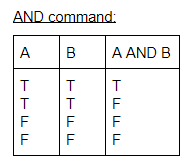Question 50
Consider the following C++ programAssuming the required header files are already included, the above program:
 A results in a compilation error B print 123 C print 111 D print 322
Programming       Operator
Question 50 Explanation:
Solution: A but official key given solution D
→ It gives compilation error because declaration of “intc” is wrong.
→ q+=b(a(q)) and r+=a(c(r)) will result compilation error.
 Question 51
A particular parallel program computation requires 100 sec when executed on a single processor. If 40% of this computation is inherently sequential (i.e. will not benefit from additional processors), then theoretically best possible elapsed times of this program running with 2 and 4 processors, respectively, are
 A 20 sec and 10 sec B 30 sec and 15 sec C 50 sec and 25 sec D 70 sec and 55 sec
Operating-Systems
Question 51 Explanation:
→ The computation requires 100 seconds on a single processor implies that 40% of the computation takes 40 seconds on any number of processors and the remaining 60% takes 60 / (#processors) seconds on parallel computation which becomes 30 seconds on two processors and 15 seconds on four.
→ Hence, in total, the computation takes 40+30=70 seconds on two processors and 40+15=55 seconds on four processors.
 Question 52
Consider the following C code segment   Of the following, which best describes the growth of f(x) as a function of x?
 A Linear B Exponential C Quadratic D Cubic
Engineering-Mathematics       Calculus
Question 52 Explanation:Question 53
For a multiprocessor architecture, In which protocol a write transaction is forwarded to only those processors that are known to possess a copy of newly altered cache line ?
 A Snoopy bus protocol B Cache coherence protocol C Directory-based protocol D None of the above
Computer-Organization
Question 53 Explanation:
→ Directory based coherence is a mechanism to handle Cache coherence problem in Distributed shared memory (DSM).
→ Non-Uniform Memory Access (NUMA). Another popular way is to use a special type of computer bus between all the nodes as a "shared bus" (System bus).
→ Directory-based coherence uses a special directory to serve instead of the shared bus in the bus based coherence protocols.
→ In directory-based cache coherence, this is done by using this directory to keep track of the status of all cache blocks, the status of each block includes in which cache coherence "state" that block is, and which nodes are sharing that block at that time, which can be used to eliminate the need to broadcast all the signals to all nodes, and only send it to the nodes that are interested in this single block.
 Question 54
Given a binary-max heap. The elements are stored in an array as 25, 14, 16, 13, 10, 8, 12. What is the content of the array after two delete operations?
 A 14,13,8,12,10 B 14,12,13,10,8 C 14,13,12,8,10 D 14,13,12,10,8
Data-Structures       Binary-Heap
Question 54 Explanation:
Step-1: Initially, the heap structure isStep-2: We have to perform 2 delete operations.
In max-heap (or) min-heap by default we are deleting root element only.
After 1st delete, the heap structure isStep-3: After 2nd delete operation, the heap structure isQuestion 55
The Functions Point (FP) metric is
 A Calculated from user requirement B Calculated from lines of code C Calculated from software complexity assessment D None of the above
Software-Engineering
Question 55 Explanation:
→ The functional user requirements of the software are identified and each one is categorized into one of five types: outputs, inquiries, inputs, internal files, and external interfaces.
→ Once the function is identified and categorized into a type, it is then assessed for complexity and assigned a number of function points.
→ Each of these functional user requirements maps to an end-user business function, such as a data entry for an Input or a user query for an Inquiry.
→ This distinction is important because it tends to make the functions measured in function points map easily into user-oriented requirements, but it also tends to hide internal functions (e.g. algorithms), which also require resources to implement.
 Question 56
The lower degree of cohesion is kind of
 A Logical Cohesion B Coincidental Cohesion C Procedural Cohesion D Communicational Cohesion
Software-Engineering       Coupling-and-Cohesion
Question 56 Explanation:
Cohesion is a measure of the degree to which elements of a module are functional related.Question 57
What is the output of the following program ?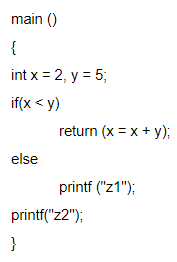A z2 B z1z2 C Compilation error D None of these
Programming-for-Output-Problems       if-else
Question 57 Explanation: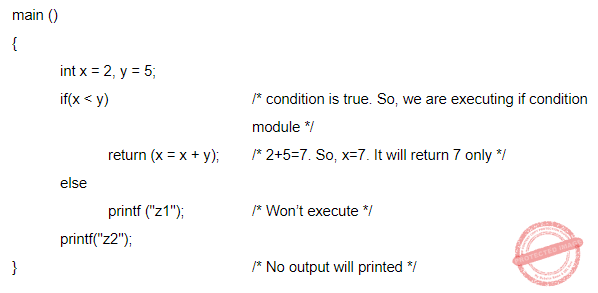Question 58
The Operating System of a computer may periodically collect all the free memory space to form contiguous block of free space. This is called:
 A Concatenation B Garbage collection C Collision D Dynamic Memory Allocation
Operating-Systems       Memory-Management
Question 58 Explanation:
→ The Operating System of a computer may periodically collect all the free memory space to form a contiguous block of free space. This is called garbage collection
→ We can also use compaction to minimize the probability of external fragmentation.
→ In compaction, all the free partitions are made contiguous and all the loaded partitions are brought together.
 Question 59
Consider the following programAssuming required header files are included and if the machine in which this program is executed is little-endian, then the output will be
 A 0 B 99999999 C 1 D unpredictable
Programming-for-Output-Problems       Pointers
Question 59 Explanation: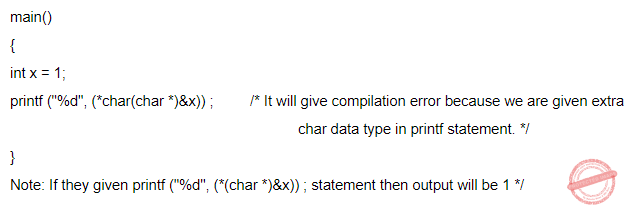Question 60
Consider the following declaration:A person.name +2 B kd → (name +2 ) C *((*kd).name + 2 ) D either (a) or (b), but not (c)
Programming-for-Output-Problems       Structure
Question 60 Explanation:
*(kd -> name +2) /* this is wrong statement */
We have to write *((*kd).name+2).
Note: They are specifically asked about a value stored at that location of the string by using "*" outside the brackets. Otherwise, option A and B would be correct.
 Question 61
If a variable can take only integral values from 0 to n, where n is an integer, then the variable can be represented as a bit field whose width is (the log in the Solutions are to the base 2, and [log n] means the floor of log n)
 A [log(n)] + 1 bits B [log (n-1)) + 1 bits C [log (n+1)] + 1 bits D None of the above
Digital-Logic-Design       Number-Systems
Question 61 Explanation:Question 62
The following C program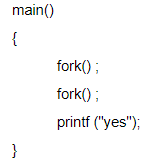If we execute this core segment, how many times the string yes will be printed ?
 A Only once B 2 times C 4 times D 8 times
Operating-Systems       System-Calls
Question 62 Explanation:
There is a direct formula to find the number of child processes is 2n -1 =3 times and already one ‘yes’ they are given. So, it will print 4 times ‘yes’
 Question 63
Given √(224)r = 13r the value of radix r is
 A 10 B 8 C 6 D 5
Digital-Logic-Design       Number-Systems
Question 63 Explanation:
√(224)r = 13r
For f(x) to be maximum
f'(x) = 4x - 2 = 0
⇒ x = 1/2
So at x = 1/2, f(x) is an extremum (either maximum or minimum).
f(2) = 2(2)2 - 2(2) + 6 = 10
f(1/2) = 2 × (1/2)2 - 2 × 1/2 + 6 = 5.5
f(0) = 6
So, the maximum value is at x=2 which is 10 as there are no other extremum for the given function.
 Question 64
Determine the number of page faults when references to pages occur in order – 1, 2, 4, 5, 2, 1, 2, 4. Assume that the main memory can accommodate 3 pages and the main memory already has the pages 1 and 2, with page 1 having brought earlier than page 2.(assume LRU algorithm is used)
 A 3 B 4 C 5 D None of these
Operating-Systems       Page-Replacement-algorithm
Question 64 Explanation:
Page fault table isThe main memory already has the pages 1 and 2, with page 1 having brought earlier than page 2. So, total 6 page faults. 6-2=4
 Question 65
Consider a system having m resources of the same type. These resources are shared by 3 processes A, B, C which have peak time demands of 3, 4, 6 respectively. The minimum value of m that ensures deadlock will never occur is
 A 11 B 12 C 13 D 14
Question 65 Explanation:
A requires 3, B-4, C-6;
→ If A has 2, B has 3, C has 5 then a deadlock will occur i.e., 2+3+5=10.
→ If we have one extra resource then a deadlock will not occur i.e., 10+1=11.
→ If we have equal (or) more than 11 resources then deadlock will never occur.
 Question 66
A computer has 1000 K of main memory. The jobs arrive and finish in the sequence Job 1 requiring 200 K arrives Job 2 requiring 350 K arrives Job 3 requiring 300 K arrives Job 1 finishes Job 4 requiring 120 K arrives Job 5 requiring 150 K arrives Job 6 requiring 80 K arrives Among the best fit and first fit, which performs better for this sequence?
 A First fit B Best fit C Both perform the same D None of the above
Operating-Systems       Memory-Management
Question 66 Explanation:
Main memory = 1000K
Job 1 requiring 200 K arrives
Job 2 requiring 350 K arrives
Job 3 requiring 300 K arrives and assuming continuous allocation:
Free memory = 1000 − 850(200 + 350 + 300) = 150 K (till these jobs first fit and best fit are same)
Since, job 1 finishes, Free memory = 200 K and 150 K
Case 1: First fit
Job 4 requiring 120 K arrives
Since 200 K will be the first slot, so Job 4 will acquire this slot only. Remaining memory = 200 – 120 = 80 K
Job 5 requiring 150 K arrives
It will acquire 150 K slot
Job 6 requiring 80 K arrives
It will occupy 80 K slot, so, all jobs will be allocated successfully.
Case 2: Best fit
Job 4 requiring 120 K arrives
It will occupy best fit slot which is 150 K. So, remaining memory = 150 − 120 = 30 K
Job 5 requiring 150 K arrives
It will occupy 200 K slot. So, free space = 200 − 150 = 50 K
Job 6 requiring 80 K arrives
There is no continuous 80 K memory free. So, it will not be able to allocate.
So, first fit is better.
 Question 67
Disk requests come to a disk driver for cylinders in the order 10, 22, 20, 2, 40, 6, and 38 at a time when the disk drive is reading from cylinder 20. The seek time is 6 ms/cylinder. The total seek time if the disk arm scheduling algorithms is first-come-first-served is360 ms
 A 360 ms B 850 ms C 900 ms D None of the above
Operating-Systems       CPU-Scheduling
Question 67 Explanation:
FCFS
Total seek time in FCFS Scheduling when the disk drive is reading from cylinder 20 for cylinders in the order 10, 22, 20, 2, 40, 6, and 38.Question 68
A hash table with 10 buckets with one slot per bucket is depicted here. The symbols, S1 to s7 are initially entered using a hashing function with linear probing. The maximum number of comparisons needed in searching an item that is not present is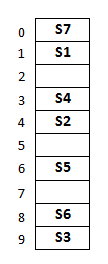A 4 B 5 C 6 D 3
Data-Structures       Hashing
Question 68 Explanation:
In this, maximum size of cluster=4(S6, S3, S7, S1)
→ Worst case of finding a number is equal to maximum size of cluster + 1(after searching all the cluster it enters into empty cluster)
→ Maximum number of comparisons = 4 + 1 = 5
 Question 69
The running time of an algorithm is given byThen what should be the relation between T(1), T(2) and T(3), so that the order of the algorithm is constant?
 A T(1) = T(2) = T(3) B T(1) + T(3) = 2 * T(2) C T(1) – T(3) = T(2) D T(1) + T(2) = T(3)
Algorithms       Time-Complexity
Question 69 Explanation:
T(n)= T(n-1) + T(n-2) – T(n-3)"
Solution:
Better way of solving is substitute.
T(1) = 1
T(2) = 2
T(3) = 3
T(4) = T(3) + T(2) - T(1) = 4
T(5) = T(4) + T(3) - T(2) = 5
:
:
T(n) = T(n-1) + T(n-2) - T(n-3) = n-1 + n-2 - n + 3 = n
So order is O(n)
 Question 70
The number of edges in a regular graph of degree d and n vertices is
 A maximum of n and d B n + d C nd D nd / 2
Engineering-Mathematics       Graph-Theory
Question 70 Explanation:
Sum of degree of vertices = 2 × no. of edges
d * n = 2 * |E|
∴ |E| = d*n/2
 Question 71
Perform window to viewport transformation for the point (20, 15). Assume that (Xwmin, Ywmin) is (0,0); (Xwmax, Ywmax) is (100,100); (Xvmin, Yvmin) is (5,5); (Xvmax, Yvmax) is (20,20). The value of x and y in the viewport is
 A x = 4 , y = 4 B x = 3 , y = 3 C x = 8 , y = 7.25 D x = 3 , y = 4
Question 71 Explanation: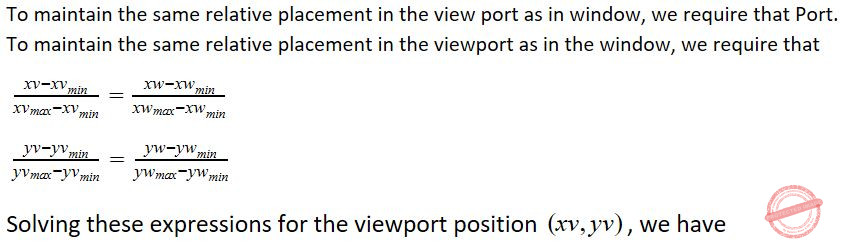Question 72
Given relations R(w,x) and S(y,z), the result of SELECT DISTINCT w, x FROM R, S Is guaranteed to be the same as R, if
 A R has no duplicates and S is non-empty B R and S have no duplicates C S has no duplicates and R is non-empty D R and S have the same number of tuples
Database-Management-System       SQL
Question 72 Explanation:
→ R has no duplicate if R can have duplicates it can be removed in the final state.
→ S in non-empty if S is empty then R*S becomes empty.
 Question 73
Database-Management-System
 A Physical Data Independence B Logical Data Independence C Both (a) and (b) D None of the above
Database-Management-System       SQL
Question 73 Explanation:
Logical data independence:
The ability to change the Conceptual (Logical) schema without changing the External schema (User View) is called logical data independence. For example, the addition or removal of new entities, attributes, or relationships to the conceptual schema or having to rewrite existing application programs.

Physical data independence:
The ability to change the physical schema without changing the logical schema is called physical data independence. For example, a change to the internal schema, such as using different file organization or storage structures, storage devices, or indexing strategy, should be possible without having to change the conceptual or external schemas.
Note: Immunity is when data at one layer is changed, it does not affect the data at another level.
 Question 74
The set of attributes X will be fully functionally dependent on the set of attributes Y if the following conditions are satisfied.
 A X is functionally dependent on Y B X is not functionally dependent on any subset of Y C Both (a) and (b) D None of these
Database-Management-System       Functional-Dependency
Question 74 Explanation:
→ A functional dependency is a constraint between two sets of attributes in a relation from a database. In other words, functional dependency is a constraint that describes the relationship between attributes in a relation.
→ An attribute is fully functional dependent on another attribute, if it is Functionally Dependent on that attribute and not on any of its proper subset.
For example, an attribute X is fully functional dependent on another attribute Y, if it is Functionally Dependent on X and not on any of the proper subset of Y.

Must satisfied conditions:
i) X is functionally dependent on Y and
ii) X is not functionally dependent on any subset of Y.
 Question 75
Let us assume that transaction T1 has arrived before transaction T2. Consider the schedule
S=r1(A); r2(B) ; w2(A); w1(B)
Which of the following is true?
 A Allowed under basic timestamp protocol. B Not allowed under basic timestamp protocols because T1 is rolled back C Not allowed under basic timestamp protocols because T2 is rolled back D None of these
Database-Management-System       Transactions
Question 75 Explanation:→ There are 2 conflicting actions a and b is shown in above diagram.
→ In timestamp ordering protocol, conflicting actions in ascending order time-stamps are allowed i.e 'a' is allowed but not 'b'.
→ So we need to roll back T1 after that only it will be allowed. Because of all conflicting actions in ascending order timestamps in below diagram.Question 76
The time complexity of computing the transitive closure of binary relation on a set of n elements is known to be
 A O(n) B O(n log n) C O(n3/2) D O(n3)
Algorithms       Time-Complexity
Question 76 Explanation:
→ In mathematics the transitive closure of a binary relation R on a set X is defined as the smallest relation on X that contains R and is transitive. More formally, the transitive closure of a binary relation R on a set X is the transitive relation R+ on set X such that R+ contains R and R+ is minimal.
→ For example, if X is a set of airports and x R y means "there is a direct flight from airport x to airport y" (for x and y in X), then the transitive closure of R on X is the relation R+ such that x R+ y means "it is possible to fly from x to y in one or more flights". Informally, the transitive closure gives you the set of all places you can get to from any starting place.
→ To find the transitive closure of given relation, we can represent the given relation by the graph such that if x R y then there should be directed edge between x and y in a graph.
→ The time complexity of floyd warshall algorithm is O(V3) where V is the number of vertices in the given graph. Take V as the number of elements is set i.e., N.
→ Therefore time complexity for the given question is O(n3).
 Question 77
In multiprogramming systems, it is advantageous if some programs such as editors and compilers can be shared by several users. Which of the following must be true of multi-programmed systems in order that a single copy of a program can be shared by several users. I. The program is a macro II. The program is recursive III. The program is reentrant
 A I only B II only C Ill only D I, II and III
Operating-Systems       Multiprogramming
Question 77 Explanation:
→ Reentrant code is commonly required in operating systems and in applications intended to be shared in multi-use systems. A programmer writes a reentrant program by making sure that no instructions modify the contents of variable values in other instructions within the program.
→ Each time the program is entered for a user, a data area is obtained which keep all the variable values for that user. The data area is in another part of memory from the program itself. When the program is interrupted to give another user a turn to use the program, information about the data area associated with that user is saved.
→ When the interrupted user of the program is once again given control of the program, information in the saved data area is recovered and the program can be re-entered without concern that the previous user has changed some instruction within the program.
 Question 78
Let P be a procedure that for some inputs calls itself (i.e. is recursive). If P is guaranteed to terminate, which of the following statement(s) must be true?
I. P has a local variable
II. P has an execution path where it does not call itself
III. P either refers to a global variable or has at least one parameter
 A I only B II only C III only D II and III only
Data-Structures
Question 78 Explanation:
In any recursive procedure:
→ P has an execution path where it does not call itself
→ P either refers to a global variable or has at least one parameter

Recursion Rules
Each recursive call should be on a smaller instance of the same problem, that is, a smaller subproblem.
The recursive calls must eventually reach a base case, which is solved without further recursion.
 Question 79
Consider the following C programHow many lines of output does this program produce?
 A 0-9 lines of output B 10-19 lines of output C 20-29 lines of output D More than 29 lines of output
Programming-for-Output-Problems       Control Flow
Question 79 Explanation:
→ Since there is one line of output for each loop, we need to determine the number of
times the loop executes. Since i is constant, we need to see the growth of j only.
→ Let the initial value of j be denoted by J1 and the subsequent values by Jn for n= 2, 3,... so that J denotes a progression of j. We see that Jn = 2Jn-1;
→ J1 = 2, which gives Jn = 2n-1;
n = 1, 2, ...
→ The loop will execute as long as i/j=2.0/2n-1 >0.001 which gives n<3log210+2 (or) n<11.97.
→ Thus the loop will execute 11 times which is equivalent to say there will be 11 lines of output.
 Question 80
An array A consists of n integers in locations A, A ….A[n-1]. It is required to shift the elements of the array cyclically to the left by k places, where 1 <= k <= (n-1). An incomplete algorithm for doing this in linear time, without using another array is given below. Complete the algorithm by filling in the blanks. Assume alt the variables are suitably declared.A i > min; j!= (n+i)mod n; A[j + k]; temp; i + 1 ; B i < min; j!= (n+i)mod n; A[j + k]; temp; i + 1; C i > min; j!= (n+i+k)mod n; A[(j + k)]; temp; i + 1; D i < min; j!= (n+i-k)mod n; A[(j + k)mod n]; temp; i + 1;
Programming       Arrays
Question 80 Explanation:
This question need presence of mind.
Step-1: Observe all options, 4 options they are given 4th and 5th blank is temp and i+1.
So, they given clue that, 4th and 5th options must be temp and i+1.
Step-2: Based on the 4th and 5th options, we can guess that 1st blank is i → Suppose if we are considering i>min then the control goes out of the while loop in the initial case when i=0 and min=n. So, 1st blank should be i Step-3: According to 1st blank, we can eliminate A and C options.
Step-4: Assume 2nd blank is correct, we are considering j!= (n+i) mod n this condition in while loop.
→ The meaning of the condition is “j becomes equal to (n+i)mod n then control goes out of the while loop.”
Step-5: The condition (n+i)mod n=i and j is always equal to i because we are assigning the value of i to j in the code segment line 3.
Step-6: based on these constraint we can say that 4th option is correct.
Reason: The control never enters the 2nd while loop. Sometimes it will enter the 2nd while loop, when we shift the numbers. It means total K places left.
There are 80 questions to complete.
PHP Code Snippets Powered By : XYZScripts.com